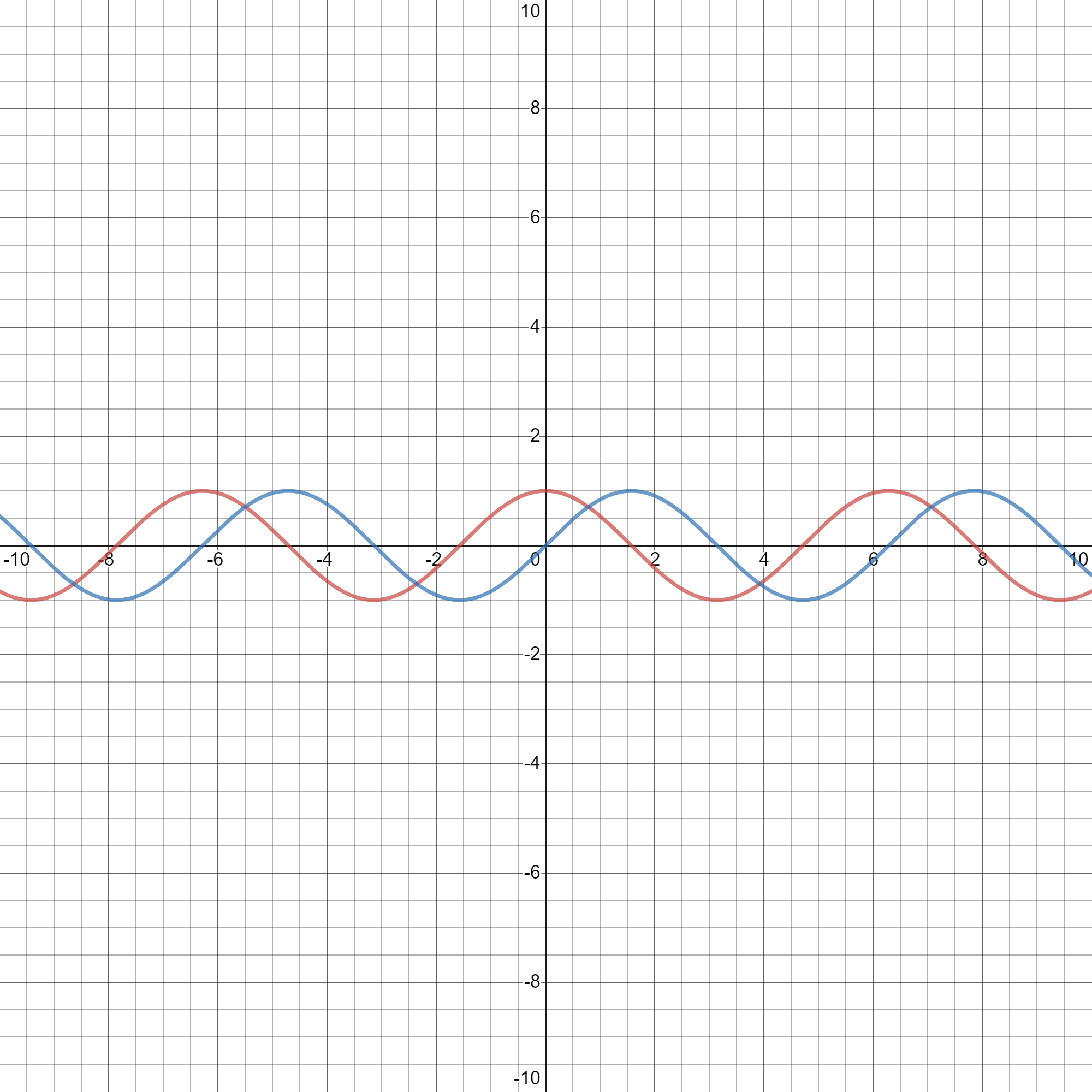# Category: Indefinite integrals## Integral of cos(x)

The integral of cos(x) is sin(x), but why? Here you’ll find the demonstration using Euler’s identity!## Integral of S(x) and C(x)

Here I talked about special functions, and at the end of the post I said I would explain how to integrate these special functions. So here I am, showing you how to integrate the functions S(x) and C(x). If you gave it a try, let me know in the comments if you used this approach! Integral of S(x) Like we did for the previous integrals, we are going to use integration by parts, as it is the only way to go. Now we can use u-substitution to solve the integral:… Read more Integral of S(x) and C(x)

## Integral of Si(x) and Ci(x)

Here I talked about special functions, and at the end of the post I said I would explain how to integrate these special functions. So here I am, showing you how to integrate the functions Si(x) and Ci(x). If you gave it a try, let me know in the comments if you used this approach! Integral of Si(x) U-substitution won’t work, so let’s use integration by parts. We know that Si(x) is given by the integral of sin(x)/x, therefore the derivative of Si(x) is sin(x)/x. Integral of Ci(x) For this… Read more Integral of Si(x) and Ci(x)## Integral of erf(x)

Here I talked about special functions, and at the end of the post I said I would explain how to integrate these special functions. So here I am, showing you… Read more Integral of erf(x)

## Integral of Ei(x)

Here I talked about special functions, and at the end of the post I said I would explain how to integrate these special functions. So here I am, showing you how to integrate the function Ei(x). If you gave it a try, let me know in the comments if you used this approach! We said that Ei(x) is equal to the integral of (e^x)/x, which means that the derivative of Ei(x) is (e^x)/x. What we need to figure out is what integration technique will actually be helpful. Honestly, u-substitution is… Read more Integral of Ei(x)

## Special functions (integrals)

U-substitution, integration by parts and trigonometric substitution are the most used techniques for integration. When nothing is going to work in the case of a certain function, we’ll say that that function doesn’t have an elementary antiderivative, which means there is no function whose derivative gives the function we want to integrate. This is why special functions have been invented. Although this looks innocent, you’ve got to believe me when I say that no matter what you do, you will never solve this. Since the antiderivative is a function we… Read more Special functions (integrals)

## Integration techniques | Trigonometric substitution

When none of the techniques you’ve learnt so far (u-substitution 1, 2,3,4 and integration by parts seem to work, you might consider trigonometric substitution. Basically, if you have a function that reminds you of a trigonometric identity, you let that function be equal to the result of the identity, for example cos²x from 1-sin²x, and then differentiate. To make things more clear I’m going to use this example: U substitution and integration by parts are not going to be very helpful. What we are gonna do instead is let x=sin(theta)… Read more Integration techniques | Trigonometric substitution

## Integration with respect to a function of x

You might think: what the hell are you talking about? I’m talking about this: Looks crazy, right? After this lesson it’s gonna look cool, trust me! Let’s calculate the integral of x with respect to x². One day I thought: “what is the integral of a function of x with respect to x²? That’s impossible I guess.” A couple of months later, that is less than a week ago, I realised: “substitution!”. If we know how to integrate with respect to x, u, t or whatever letter it is, then… Read more Integration with respect to a function of x

{{#pages}} {{/pages}}
%%footer%%